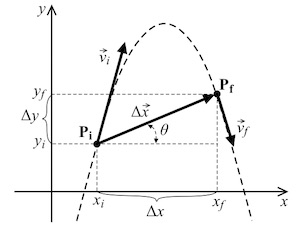Physics Department | Lehigh UniversityTeaching

## PHY 10: General Physics 1

Fall Semester

Initial Competences Required for this course (what you should know already)
Before starting this course, students should already be able to do simple operations with vectors (including adding and subtracting vectors, scalar product, and vector product) and be able to use basics calculus (derivatives and integrals). Physics 10 requires a little less use of calculus compared to the course taken by engineering and physics majors (PHY 11), still you will need to use integrals and derivatives in some very simple applications. Some of you may also need to get back in shape with writing and solving algebraic equations: Do not worry if you will struggle the first couple of weeks with algebra, by seriously working at the homework you will automatically gain back your skills!

Course contents (what will be taught in this course)
Physics 10 is the first part of General Physics (General Physics I, General Physics II is taught in Physics 13). Physics 10 is a calculus based introductory course to physics, and it is meant mainly for students oriented for example towards the biological and environmental sciences, or pre-med students. Subjects covered include mechanics, energy, momentum, thermodynamics, and fluids. The purpose of this course is to introduce these topics, and learn to apply their underlying principles to the solution of concrete problems. Starting with simple problems, you will learn to develop strategies for solving more and more complex ones.

Competences expected after this courseÊ (what you will be able to do when done)
After this course, students should be able to analyze both conceptually and quantitatively various situations encountered in physics. At a minimum, they will be able to:

• calculate and predict the motion of projectiles, or rotating objects
• critically analyze situations of equilibrium and non-equilibrium, for translational and rotational motion, using NewtonÕs Laws (with and without friction)
• set up equations based on energy conservation as applied to various kinds of situations involving different kinds of forces (from pulleys and weights to the oscillatory motion of springs and penduli, with and without friction).
• use momentum conservation to analyze different systems involving one and two-dimensional collisions between massive objects or the rotation of a solid body with changing moment of inertia. Included here is also the skill of discerning when to use or not to use energy conservation.
• analyse the physics of fluids in containers or flowing through pipes using the equation of continuity and BernoulliÕs equation, including Archimedes principle.
• analyse, using the laws of thermodynamics, pressure-volume diagrams for an ideal gas, and the behavior of thermal machines.

Your performance will not only depend on how well you are able to acquire new physics concepts, but also on your ability to solve more and more complex problems. This last point is something you will learn by solving a lot of practical physics problems.

Back to teachingContact | Goto Top of Page